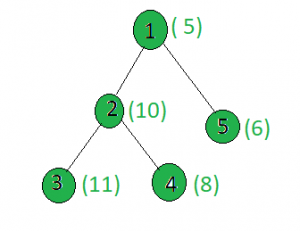Open In App

# Find the node whose absolute difference with X gives minimum value

Given a tree, and the weights of all the nodes and an integer x, the task is to find a node i such that |weight[i] – x| is minimum.
Examples:

Input:x = 15
Output:
Node 1: |5 – 15| = 10
Node 2: |10 – 15| = 5
Node 3: |11 -15| = 4
Node 4: |8 – 15| = 7
Node 5: |6 -15| = 9

Approach: Perform dfs on the tree and keep track of the node whose weighted absolute difference with x gives the minimum value.
Below is the implementation of the above approach:

## C++

 `// C++ implementation of the approach``#include ``using` `namespace` `std;` `int` `minimum = INT_MAX, x, ans;` `vector<``int``> graph;``vector<``int``> weight(100);` `// Function to perform dfs to find``// the minimum value``void` `dfs(``int` `node, ``int` `parent)``{``    ``// If current value is less than``    ``// the current minimum``    ``if` `(minimum > ``abs``(weight[node] - x)) {``        ``minimum = ``abs``(weight[node] - x);``        ``ans = node;``    ``}``    ``for` `(``int` `to : graph[node]) {``        ``if` `(to == parent)``            ``continue``;``        ``dfs(to, node);``    ``}``}` `// Driver code``int` `main()``{``    ``x = 15;` `    ``// Weights of the node``    ``weight = 5;``    ``weight = 10;``    ``weight = 11;``    ``weight = 8;``    ``weight = 6;` `    ``// Edges of the tree``    ``graph.push_back(2);``    ``graph.push_back(3);``    ``graph.push_back(4);``    ``graph.push_back(5);` `    ``dfs(1, 1);` `    ``cout << ans;` `    ``return` `0;``}`

## Java

 `// Java implementation of the approach``import` `java.util.*;``import` `java.lang.*;` `class` `GFG``{` `    ``static` `int` `minimum = Integer.MAX_VALUE, x, ans;` `    ``@SuppressWarnings``(``"unchecked"``)``    ``static` `Vector[] graph = ``new` `Vector[``100``];``    ``static` `int``[] weight = ``new` `int``[``100``];` `    ``// This block is executed even before main() function``    ``// This is necessary otherwise this program will``    ``// throw "NullPointerException"``    ``static``    ``{``        ``for` `(``int` `i = ``0``; i < ``100``; i++)``            ``graph[i] = ``new` `Vector<>();``    ``}` `    ``// Function to perform dfs to find``    ``// the minimum xored value``    ``static` `void` `dfs(``int` `node, ``int` `parent)``    ``{` `        ``// If current value is less than``        ``// the current minimum``        ``if` `(minimum > Math.abs(weight[node] - x))``        ``{``            ``minimum = Math.abs(weight[node] - x);``            ``ans = node;``        ``}``        ``for` `(``int` `to : graph[node])``        ``{``            ``if` `(to == parent)``                ``continue``;``            ``dfs(to, node);``        ``}``    ``}` `    ``// Driver Code``    ``public` `static` `void` `main(String[] args)``    ``{``        ``x = ``15``;` `        ``// Weights of the node``        ``weight[``1``] = ``5``;``        ``weight[``2``] = ``10``;``        ``weight[``3``] = ``11``;``        ``weight[``4``] = ``8``;``        ``weight[``5``] = ``6``;` `        ``// Edges of the tree``        ``graph[``1``].add(``2``);``        ``graph[``2``].add(``3``);``        ``graph[``2``].add(``4``);``        ``graph[``1``].add(``5``);` `        ``dfs(``1``, ``1``);` `        ``System.out.println(ans);``    ``}``}` `// This code is contributed by SHUBHAMSINGH10`

## Python3

 `# Python3 implementation of the approach``from` `sys ``import` `maxsize` `# Function to perform dfs to find``# the minimum value``def` `dfs(node, parent):``    ``global` `minimum, graph, weight, x, ans` `    ``# If current value is less than``    ``# the current minimum``    ``if` `minimum > ``abs``(weight[node] ``-` `x):``        ``minimum ``=` `abs``(weight[node] ``-` `x)``        ``ans ``=` `node` `    ``for` `to ``in` `graph[node]:``        ``if` `to ``=``=` `parent:``            ``continue``        ``dfs(to, node)` `# Driver Code``if` `__name__ ``=``=` `"__main__"``:``    ``minimum ``=` `maxsize``    ``graph ``=` `[[] ``for` `i ``in` `range``(``100``)]``    ``weight ``=` `[``0``] ``*` `100``    ``x ``=` `15``    ``ans ``=` `0` `    ``# Weights of the node``    ``weight[``1``] ``=` `5``    ``weight[``2``] ``=` `10``    ``weight[``3``] ``=` `11``    ``weight[``4``] ``=` `8``    ``weight[``5``] ``=` `6` `    ``# Edges of the tree``    ``graph[``1``].append(``2``)``    ``graph[``2``].append(``3``)``    ``graph[``2``].append(``4``)``    ``graph[``1``].append(``5``)` `    ``dfs(``1``, ``1``)` `    ``print``(ans)` `# This code is contributed by``# sanjeev2552`

## C#

 `// C# implementation of the approach``using` `System;``using` `System.Collections.Generic;``    ` `class` `GFG``{` `static` `int` `minimum = ``int``.MaxValue, x, ans;` `static` `List> graph = ``new` `List>();``static` `List<``int``> weight = ``new` `List<``int``>();` `// Function to perform dfs to find``// the minimum value``static` `void` `dfs(``int` `node, ``int` `parent)``{``    ``// If current value is more than``    ``// the current minimum``    ``if` `(minimum > Math.Abs(weight[node] - x))``    ``{``        ``minimum = Math.Abs(weight[node] - x);``        ``ans = node;``    ``}``    ``for` `(``int` `i = 0; i < graph[node].Count; i++)``    ``{``        ``if` `(graph[node][i] == parent)``            ``continue``;``        ``dfs(graph[node][i], node);``    ``}``}` `// Driver code``public` `static` `void` `Main(String []args)``{``    ``x = 15;` `    ``// Weights of the node``    ``weight.Add(0);``    ``weight.Add(5);``    ``weight.Add(10);;``    ``weight.Add(11);;``    ``weight.Add(8);``    ``weight.Add(6);``    ` `    ``for``(``int` `i = 0; i < 100; i++)``    ``graph.Add(``new` `List<``int``>());` `    ``// Edges of the tree``    ``graph.Add(2);``    ``graph.Add(3);``    ``graph.Add(4);``    ``graph.Add(5);` `    ``dfs(1, 1);` `    ``Console.WriteLine( ans);``}``}` `// This code is contributed by shubhamsingh10`

## Javascript

 ``

Output:

`3`

Complexity Analysis:

• Time Complexity : O(N).
In dfs, every node of the tree is processed once and hence the complexity due to the dfs is O(N) if there are total N nodes in the tree. Therefore, the time complexity is O(N).
• Auxiliary Space : O(1).
Any extra space is not required, so the space complexity is constant.